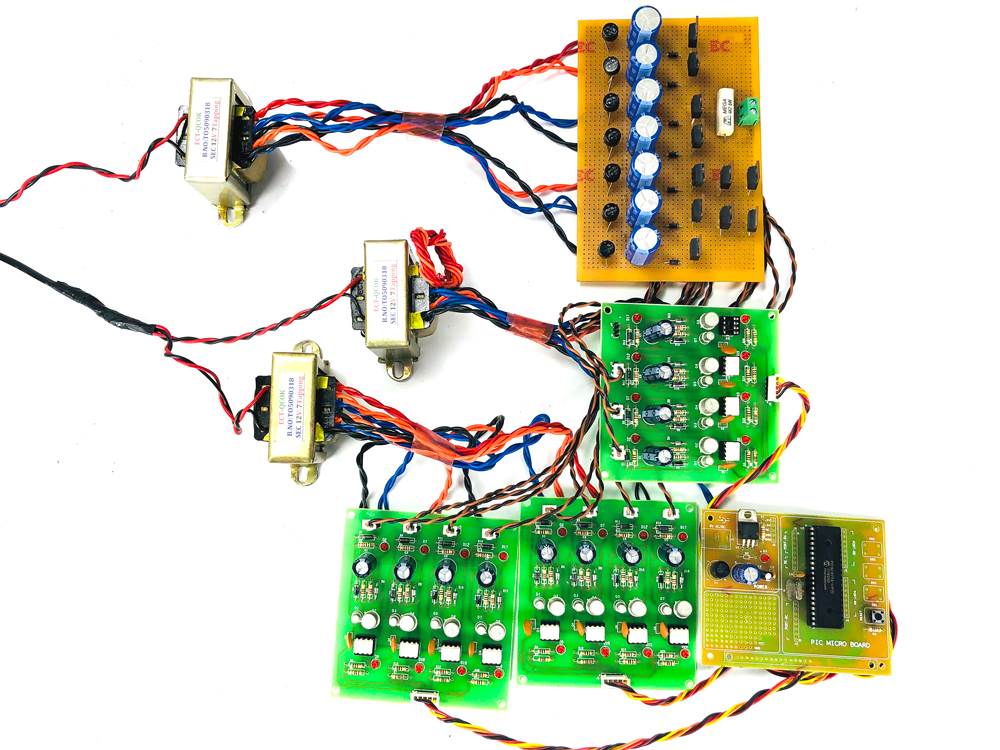## Multilevel Inverter

• Post author:
• Post category:Uncategorized

The multilevel inverter generates multiple step voltage waveform with variable and controllable frequency, phase and amplitude. This Inverter is used for high power and power quality demanding applications. The number of step will be increased in the output, the losses will be reduced. The different types of Multilevel inverters are shown below.

The cascaded multilevel inverter is known as two or more inverter outputs are connected in series connection. And produce the multilevel output range depends on the input voltage ranges (+2v, +v, 0, -v, -2v). This cascaded Multilevel Inverter has different types. There are,

### 1. Five Level Cascaded Multilevel Inverter

This five level cascaded multilevel inverter output waveform is obtain by using the two h-bridge inverters. And this h-bridge inverters are connected in cascade connection.

### 2. Seven Level Cascaded Multilevel Inverter

The seven level output is produced this novel seven level multilevel inverter by using PIC controller. This multilevel inverter is producing the seven level of output.

### 3. Nine Level Cascaded Multilevel Inverter

In normal nine level inverter needs 4 H-bridge inverters. For this nine level multilevel inverter needs 2 inverters only. So number of switches will be reduced, so switching losses will be reduced.

## 2. ﻿DSP Based Multilevel Inverter﻿

The DSP based multilevel inverter is producing seven level multilevel output by using DSP controller. The DSP controller is used to generate the PWM signals.

## 3. Fifteen Level Cascaded Multilevel Inverter

This project is produce the fifteen level output using three h- bridge inverter. The fifteen level inverter produce 15 level so harmonics will be reduced and power switches will be reduced.

## 4. Transformer Based Multilevel Inverter

This project can be produce nine level output by using transformer topology. It consists of two H-bridges fed from single DC source. It producing nine level AC output waveform.

## 5. Five Level Quasi Z-Source Multilevel Inverter

This project has two h-bridge inverter. The input voltage is boosted by using the quasi z source circuit. Boosted dc voltage is applied to the inverter circuit, this project is used for renewable energy sources.

## 6. Arduino Based Multilevel Inverter

The Arduino based multilevel inverter is producing the seven level output waveform. The Arduino controller is used to generate the PWM pulses and applied to driver circuit. The driver circuit is isolate and amplify the input signal.

## 7. Novel Seven Level Inverter

This seven level inverter is produce the seven level output by using DSP controller ( TMS320F2812 Starter Kit ). This DSP controller is used to generate the PWM pulses for this project.